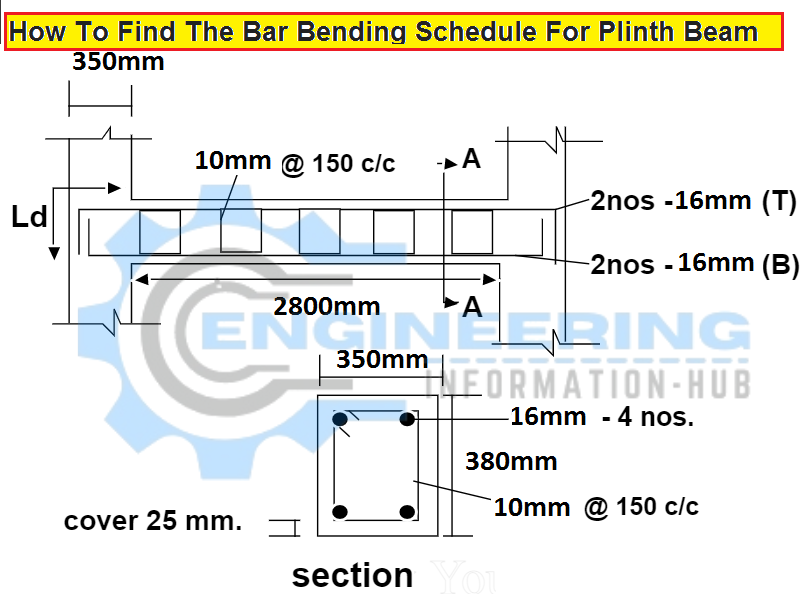# Find The Bar Bending Schedule For Plinth Beam

## Find The Bar Bending Schedule For Plinth Beam

Today I have the topic of bar bending schedule for plinth beam | what is the plinth beam| purpose of plinth beam How to calculate the bar bending schedule for plinth beam| Find The Bar Bending Schedule For Plinth Beam

### What is the plinth beam?

The plinth beam is the RCC beam that is constructed between the wall and its foundation, The main and basic purpose of the plinth beam is to prevent cracks from the foundation to the wall when the soil bearing capacity is low and settlements of soil.

### Given dataLength of beam b/w column = 2800mm = 2.8m

Width of beam = 350mm =0.350m

Thicknes of beam= 380mm=0.380m

Diameter of horizontal / straight bar =16mm

Numbers of horizontal / straight bar=04Nos

Diameter of stirrups = 10mm

Concrete cover of beam = 25mm

Spacing of stirrups = 150mm

Development length = Ld=40d=40 x 16 =640mm

90º bend =2d=2 x 16= 32mm  ( for straight bar)

90º bend=2d=2 x 10 =20mm  ( for stirrups )

135 º bend = 3d =3 x 10 =30mm ( for stirrups )

Hooks length = 10d =10 x 10 =100mm

### Horizontal of straight bar dia=16mm

Cutting length of horizontal /straight bar = Length of beam b/w column +2(Ld)-2bends (90 degree)

Putting the value

Cutting length of horizontal /straight bar =2800 + 2 (640) -2(32)

Cutting length of horizontal of straight bar =2800+1280-64=4016mm=4.016m

Total length of horizontal /straight bar = length of one bar x No’s of bar

Total length of horizontal /straight bar =4.016 x 4 =16.064m

### Wight of Horizontal of straight bar

Wight of Horizontal /straight bar = d²/162.25 x total length

Wight of Horizontal /straight bar =(16)²/162.25 x 16.064 = 25.354kg= 2kg

### Stirrups dia=10mm

Cutting length of stirrups = 2 (A+B)  + 2 hooks – 3( 90degree beds ) – 2(35 degree bends)

Now

A= width of beam – 2 concrete cover = 350 -50=300mm

B = Thickness of beam – 2 concrete cover =380 – 50 330mm

Putt the value

### Number of stirrups

Number of stirrups = total length of beam /spacing +1

Number of stirrups =2800/150+1 =19.66 = 20 No’s

The total length of stirrups = length of one stirrup x Number of stirrups

Total length of stirrups =1.400  x 20 =28m

### Wight of Stirrups

Wight of stirrups = d²/162.25 x total length

Wight of stirrups =(10)²/162.25 x 28 = 17.257 Kg = 18Kg